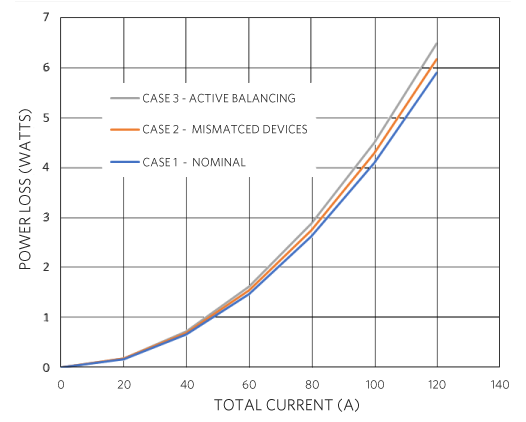# Understanding the Load-Sharing or Current-Balance Characteristics of the MAX16545 and MAX16543 Power Protection Solution

### Abstract

A common customer question about the MAX16545 and MAX16543 power-protection solution concerns the “balance” of load current between the two devices. This application note describes the load-sharing characteristics of this solution.

#### Introduction

The MAX16545 protection device can handle up to 60A of load current. To support control and protection of a +12V supply with a load current above 60A, it is necessary to combine the MAX16545 master device with one or two MAX16543 follower devices. Each MAX16543 provides an additional 30A of load-current capability, offering scaled solutions of 90A and 120A.

The balance of the load current between the two devices is not actively maintained and depends solely on the relative on-resistance of the two devices in parallel. Extraneous resistances inherent in the circuit-board construction also influence the current distribution in a predictable manner.

#### Current Balance Equation

Neglecting the effects of copper resistance in the circuit board assembly, the balance of the load current through each device is determined as follows:

MAX16545 current = IM = ILOAD × RF/(RM + RF)
MAX16543 current = IF = ILOAD × RM/(RM + RF)

RM represents the on-resistance of the MAX16545, and RF represents the resistance of the MAX16543.

The board layout resistances for the MAX16545 and the MAX16543 are represented by RMCu and RFCu respectively, as shown in Figure 1.Figure 1. Parasitic resistances with MAX16545 and MAX16543 in parallel.

The equations are as follows:

MAX16545 current = IM = ILOAD × (RF +RFCu)/(RM + RF + RFCu + RMCu)
MAX16543 current = IF = IF = ILOAD × (RM +RMCu)/(RM + RF + RFCu + RMCu)

There is no active balancing or modulation of the load current. As with any parallel n-channel, MOSFET, power-switching circuit, there is some inherent balancing due to the positive temperature coefficient-of-resistance of the MOSFET switch. If one switch is carrying more current, the on-resistance increases and forces the balance to shift away from that switch, and vice versa.

Typically, the on-resistance, RM, of the MAX16545 master is about 0.9mΩ, and the on-resistance, RF, of the MAX16543 follower is about 1.5mΩ. So, the current is nominally distributed 5 parts to 3 parts (62.5% and 37.5%) between the master and follower.

Note that both silicon and copper have a positive temperature coefficient of resistance. If a path in a multi-path parallel circuit receives more current, the heat from the resistive losses raises the temperature in that path because the resistive power loss varies with the square of the current. This causes an increase in resistance and a beneficial redistribution of current away from this path.

#### Why Not Actively Balance the Load Current?

At first glance, it might seem logical or desirable to actively control the balance of current through the devices, for example to achieve an ideal 2:1 relationship. However, to alter the balance of the load current at least one of the two devices must reduce its gate-drive voltage and, thus, increase its on-resistance (RDS,ON) to redistribute the current. This means that the overall system operates at less than maximum efficiency with no real benefit to the overall application.

Another way to think of this is that the best device must be detuned to match the worst device to perfectly balance the current between the two similar devices. This means sacrificing some performance.

This makes little sense for similar devices that have the same nominal on-resistance, and even less sense when the devices are dissimilar, as with the approximate 0.9mΩ master and 1.5mΩ follower on-resistances. Any ratio of balancing between dissimilar devices becomes arbitrary and is always fundamentally wasteful of energy.

#### Efficiency Comparison Between Passive and Active Current Distribution

Consider a three-leg circuit that uses one MAX16545 master device and two MAX16543 follower devices to provide 120A total current capability.

The hypothetical on-resistances of the devices in our circuit are as follows:

• MAX16545 fully-enhanced RDS(ON) = 0.9mΩ
• MAX16543 #1 fully-enhanced RDS(ON) = 1.8mΩ
• MAX16543 #2 fully-enhanced RDS(ON) = 1.5mΩ

With simple current-divider math, the distribution at any load current is as follows:

• MAX16545: (1.5mΩ || 1.8mΩ)/(1.5mΩ || 1.8mΩ) + 0.9mΩ) = 47.6%
• MAX16543 #1: (0.9mΩ || 1.5mΩ)/(0.9mΩ || 1.5mΩ) + 1.8mΩ) = 23.8%
• MAX16543 #2: (0.9mΩ || 1.8mΩ)/(0.9mΩ || 1.8mΩ) + 1.5mΩ) = 28.6%

As expected, there is a slight imbalance in current between the two MAX16543 followers. In the perfect case, the current through the two MAX6543 devices is equal, at roughly 27.3% each.

But what effect does this have on efficiency? The power loss is the product of the square of the load current and the parallel resistance of all pass-FET elements.

Plot the power-loss versus load-current for the following cases:

• Case 1: Perfect devices, no matching or balancing required
• Case 2: Passive matching of imperfect devices
• Case 3: Active matching, by increasing RDS(ON) of the better follower device

Figure 2 shows the power-loss for each case, plotted on the same axes. Note that the losses are highest when the follower devices are actively balanced to have the same load current.Figure 2. Power losses with and without current-balancing.

#### Conclusions

• The distribution of load current between pass devices is easy to determine and follows basic electrical engineering and physics concepts.
• It does not matter if the resistance mismatch arises from the device itself or from an imbalanced layout (i.e., parasitic copper-resistance effects).
• Higher resistance is always bad for performance, so the designer should strive for the best possible placement and layout.
• The positive temperature coefficient of resistance in silicon and copper has an inherent beneficial contribution to current distribution.
• Active balancing always makes efficiency worse because only the best-performing devices can be modulated to match the worst-performing device.
• There is nothing to be gained by attempting to actively balance current in a multi-path (parallel) resistive circuit.